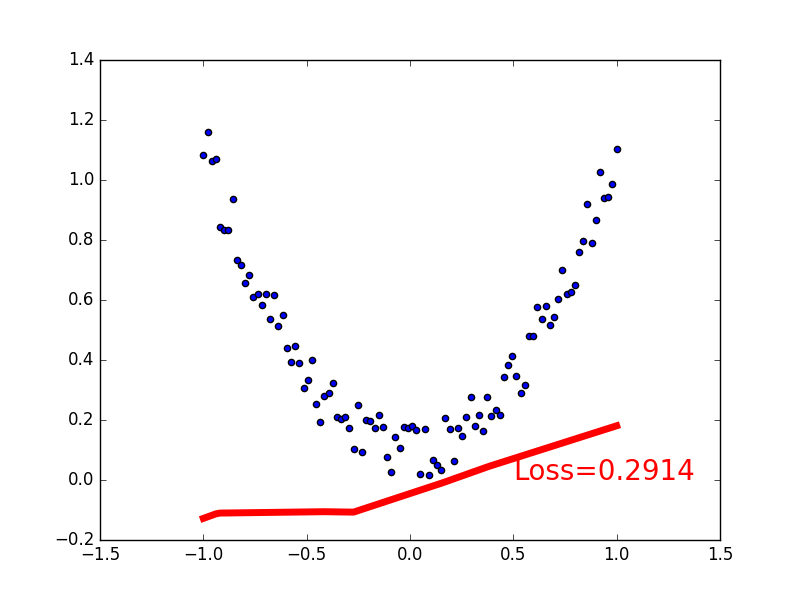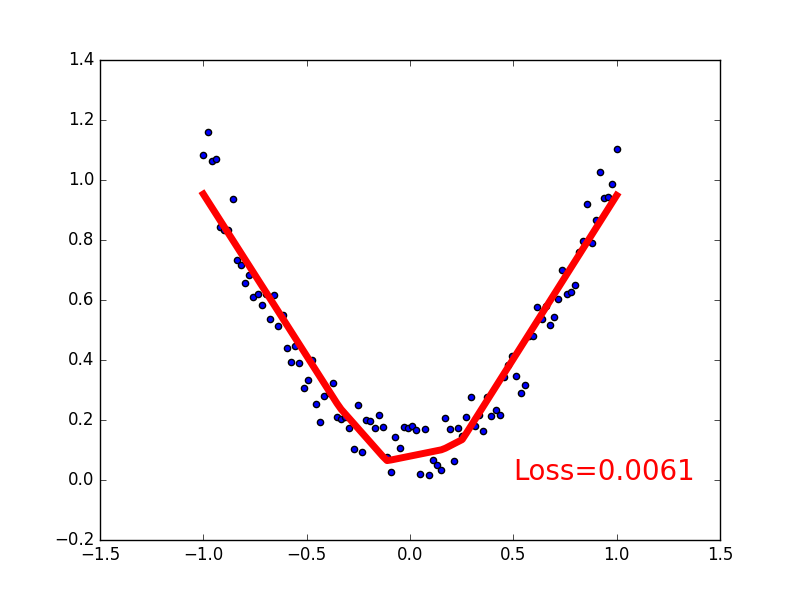# 关系拟合 (回归)

## 要点 ¶## 建立数据集 ¶

import torch
import matplotlib.pyplot as plt

x = torch.unsqueeze(torch.linspace(-1, 1, 100), dim=1)  # x data (tensor), shape=(100, 1)
y = x.pow(2) + 0.2*torch.rand(x.size())                 # noisy y data (tensor), shape=(100, 1)

# 画图
plt.scatter(x.data.numpy(), y.data.numpy())
plt.show()


## 建立神经网络 ¶

import torch
import torch.nn.functional as F     # 激励函数都在这

class Net(torch.nn.Module):  # 继承 torch 的 Module
def __init__(self, n_feature, n_hidden, n_output):
super(Net, self).__init__()     # 继承 __init__ 功能
# 定义每层用什么样的形式
self.hidden = torch.nn.Linear(n_feature, n_hidden)   # 隐藏层线性输出
self.predict = torch.nn.Linear(n_hidden, n_output)   # 输出层线性输出

def forward(self, x):   # 这同时也是 Module 中的 forward 功能
# 正向传播输入值, 神经网络分析出输出值
x = F.relu(self.hidden(x))      # 激励函数(隐藏层的线性值)
x = self.predict(x)             # 输出值
return x

net = Net(n_feature=1, n_hidden=10, n_output=1)

print(net)  # net 的结构
"""
Net (
(hidden): Linear (1 -> 10)
(predict): Linear (10 -> 1)
)
"""


## 训练网络 ¶

# optimizer 是训练的工具
optimizer = torch.optim.SGD(net.parameters(), lr=0.2)  # 传入 net 的所有参数, 学习率
loss_func = torch.nn.MSELoss()      # 预测值和真实值的误差计算公式 (均方差)

for t in range(100):
prediction = net(x)     # 喂给 net 训练数据 x, 输出预测值

loss = loss_func(prediction, y)     # 计算两者的误差

loss.backward()         # 误差反向传播, 计算参数更新值
optimizer.step()        # 将参数更新值施加到 net 的 parameters 上


## 可视化训练过程 ¶

import matplotlib.pyplot as plt

plt.ion()   # 画图
plt.show()

for t in range(200):

...
loss.backward()
optimizer.step()

# 接着上面来
if t % 5 == 0:
# plot and show learning process
plt.cla()
plt.scatter(x.data.numpy(), y.data.numpy())
plt.plot(x.data.numpy(), prediction.data.numpy(), 'r-', lw=5)
plt.text(0.5, 0, 'Loss=%.4f' % loss.data.numpy(), fontdict={'size': 20, 'color':  'red'})
plt.pause(0.1)